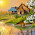## Saturday, June 18, 2011

### Temperature sensor with PIC18 and MCP9700

This can be a complete project on its own - a simple DIY digital thermometer with LCD display and only a handful of parts - the PIC18F45K20, LM35 and a small number of resistors and capacitors running off a regulated 5v supply.

Display type - LCD (can be 16x1, 16x2 or anything larger)
Controller: PIC18F45K20
Programming Language: BASIC
Compiler: mikroBASIC PRO for PIC v3.20

'''''''''''''''''''''''''''''''''''''''''''''''''''''''''''''''''''''''''''''''''''''''''''''''''''''''''''''''''''''''''''''''''''''''''''''''''''''''''''''''''''''''''''
'Programmer: Syed Tahmid Mahbub
'Compiler: mikroBASIC PRO for PIC v3.20
'Target PIC: 18F45K20

program MC9700_Temp

dim LCD_RS as sbit at RC4_bit
LCD_EN as sbit at RC5_bit
LCD_D4 as sbit at RC0_bit
LCD_D5 as sbit at RC1_bit
LCD_D6 as sbit at RC2_bit
LCD_D7 as sbit at RC3_bit

LCD_RS_Direction as sbit at TRISC4_bit
LCD_EN_Direction as sbit at TRISC5_bit
LCD_D4_Direction as sbit at TRISC0_bit
LCD_D5_Direction as sbit at TRISC1_bit
LCD_D6_Direction as sbit at TRISC2_bit
LCD_D7_Direction as sbit at TRISC3_bit

dim Temp as longword
dim vDisp as word
dim Display as string

main:
TRISA = \$FF
TRISC = 0
PORTC = 0
LCD_Init()
LCD_Cmd(_LCD_CURSOR_OFF)
LCD_Cmd(_LCD_CLEAR)
LCD_Out(1, 1, "Temp:")
Display = "+125 'C"
while true
Temp = (100 * ( (10 * ADRead) - 1024 ) ) >> 11 'Get temperature
Display = "+"
else 'If temperature is negative
Temp = ( (1024 - (10 * ADRead) ) * 100 ) >> 11 'Get temperature
Display = "-"
end if
vDisp = Temp div 100
vDisp = (Temp div 10) mod 10
vDisp = Temp mod 10
Display = vDisp + 48
Display = vDisp + 48
Display = vDisp + 48
LCD_Out(1, 8, Display) 'Show temperature
wend
end.http://www.4shared.com/file/b8bT5a9e/MC9700_temperature_sensor.html

The code can be freely used and there is no copyright restrictions or anything as such. Only credit the author (me) where necessary.

1.2.